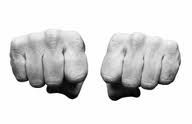Ask a friend to hide a 5c coin in one hand and a 10c coin in the other without showing you which coin is in which hand. Then say to  your friend:

“Multiply the amount of money in your left hand by 2 or 4 (whichever you like) and the amount of money in your right hand by 3 or 5 (whichever you like), then add the results and tell me the total.”

According to whether the answer given is even or odd you can tell which coin was in which hand.

Can you explain how and why this trick works?

Thanks to The Mathematical Digest (click here) for this trick.

Notes for Teachers – Which Hand?

### 5 Responses to Grades 8 to 12 Which Hand?

1.toni says:

This problem gives practice in mental arithmetic. The maths needed is multiplying by 2, 3, 4 and 5 and adding – all primary school work. To understand the trick you just need to appreciate the properties of odd and even numbers.

2.Mr 'H' Hempe says:

The problem is simple but interesting. Learners should know the properties of even and odd numbers.After trying this exercise a few times learners should be able to see the pattern.Learners should know that adding an odd number to an even number gives you an odd number, and multiplying any number by 2 or 4 (which are both even) will give you an even number.

3.Jalamba Mjulwa says:

I gave my grade 12 class this question to do they enjoy to do without knowing the aim of it, after a some i ask them a questions like what they notice to this experiment really its easy to tell. Really here its only understanding of even numbers and odd numbers.

4.Jalamba Mjulwa says:

So that each and every one know what exactly happen do the experiment and record the findings you will see what is happening

5.Tau Tamako says:

I gave the activity on ” which hand” to my grade 09 learners and found it to be a very good mental maths activity which can be given to learners in different grades.